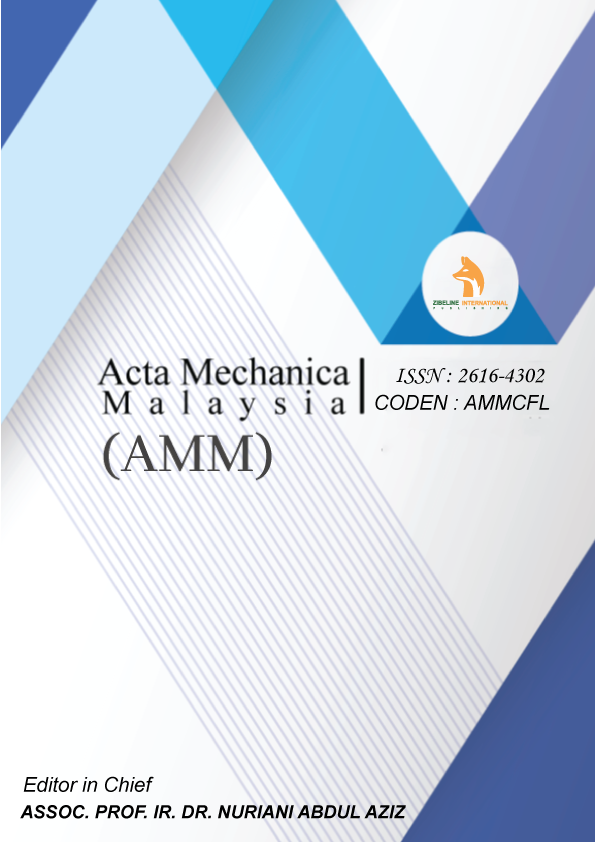##### AN EFFICIENT PSEUDO-POTENTIAL MULTIPHASE LATTICE BOLTZMANN SIMULATION MODEL FOR THREE-DIMENSIONAL MULTIPHASE FLOWS

ABSTRACT

In this paper, a single-component pseudo-potential model is analyzed by modifying corresponding equation of state. Simulation results show that with the modified vdW equation, this model is capable to simulate the multiphase flow with greater range of density ratio than other existing multiphase models and some drawbacks of pseudo-potential model such as large spurious currents are avoided as well. Besides, the influences of the modified method on surface tension are studied. Also, two other typical equations of state are employed, and the simulation results show that both of them work very well under any characteristic temperature and the greatest density ratio could be up to 109.

KEYWORDS

Lattice Boltzmann model, Multiphase, Pseudo-potential model, Large density ratio